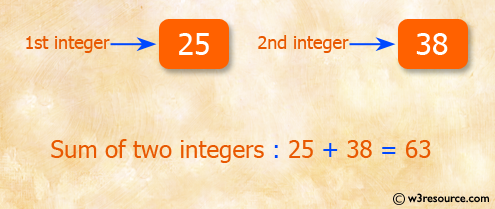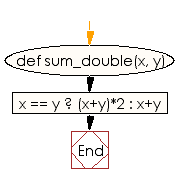﻿ Ruby Basic exercises: Compute the sum of the two integers - w3resource# Ruby Basic Exercises: Compute the sum of the two integers

## Ruby Basic: Exercise-22 with Solution

Write a Ruby program to compute the sum of the two integers, if the two values are equal return double their sum otherwise return their sum.Ruby Code:

``````def sum_double(x, y)
x == y ? (x+y)*2 : x+y
end

print sum_double(5, 5),"\n"
print sum_double(4, 5)
``````

Output:

```20
9
```

Flowchart:Ruby Code Editor: